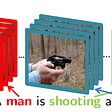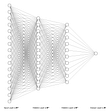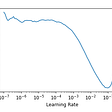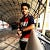# A VLSI DESIGN OF THE MINIMUM ENTROPY NEURON

In pattern recognition, the maximization of the transformation from the input to the output of the system corresponds to minimize the mean square error of the predicted output. For linear models, it is done by linear transformations. Generally, we encode the input data by using only the eigenvectors with the biggest eigenvalues. Such neural networks use linear neurons. They use a basic building block the linear neuron. That neuron learns or the input weights are optimized by a Hebb-rule. We can get the input for each stage by subtracting sequentially all the outputs of weight vectors. In learning mode, the input is forwarded sequentially through several neurons. Whereas in transforming mode, the input is forwarded to the neurons in parallel as shown in Fig 1.

An important key feature of the VLSI design paradigm in fast and real-time based architecture. For neural networks, it is better and efficient to use parallel processors to perform operations simultaneously in parallel. Fig 1 illustrates this system, as the input data is forwarded to the next neurons in parallel via the input bus.

To design the weights of the neural network by voltages wij capacitors Cij, each input xj is primarily multiplied by weight w in the MUL module, then the obtained output is summed in SUM modules. The learning of the network or optimizing the weights is performed in the HEBB module and the NORM module is used for normalization. The design paradigm of the single neuron is shown in Fig. 2

For multiplication in the MUL block, the well-known gilbert four quadrant multiplier circuit should be used. Also for multiplications in the HEBB module, this multiplier circuit should be used. For designing the SUM block (implement the sum), we shouldn’t use the virtual ground circuit. Instead of that, we should use a simple current adder with an amplifier. This circuit is illustrated in Fig. 3.

To reduce the requirements of resources, the normalization block can be implemented with only three non-linear circuits. As shown in fig. 4, the normalization block should be designed in such a way that the weights signals should be transformed in square waves, and then a proportional current should be induced. After that, the relative current in several circuit channels is balanced according to the relative values of the input signal. These currents should be read out and backpropagated to the input and then we will get the normalized weights.

In this way, the minimum entropy neuron can be implemented with an analog VLSI design.

References

1. Oja, E., “A Simplified Neuron Model as a Principal Component Analyzer”, J. Math. Biol. vol 13, pp.267–273, 1982

2. RüdigerW. Brause, “A Vlsi Design of the Minimum Entropy Neuron”, VLSI for Neural Networks and Artificial Intelligence, pp. 53–60, 1994.

--

--

--

## More from VLSI for Neural Networks and Artificial Intelligence

Neural Network Model Deployment on VLSI Devices

## Is Deep Learning Smart Enough to Generate Fake News?## 5 things you need to know about Logistic Regression## Describing Videos by Exploiting Temporal Structure## Emergency Chatbot using Rasa on Jupyter Notebook/Google Colaboratory## Classifying Handwritten Digits Using A Multilayer Perceptron Classifier (MLP)## The Best Uses of TensorFlow API Ever!## Improving Our Model## A Primer on Reinforcement Learning## Permission Granted## 6.9.2022## Meet Elizabeth Hamilton-Guarino, the Chief Executive Officer of Compliance4, as well as the founder…